+ Text Only Site
+ Non-Flash Version
+ Contact Glenn## Weight and Balance Forces Acting on an Airplane

SUBJECT: Physics
TOPIC: Torques and Moments
DESCRIPTION: A set of mathematics problems dealing with torques and moments.
CONTRIBUTED BY: Carol Hodanbosi
EDITED BY: Jonathan G. Fairman - August 1996

#### Principle: Balance of forces produces Equilibrium

Gravity always acts downward on every object on earth. Gravity multiplied by the object's mass produces a force called weight. Although the force of an object's weight acts downward on every particle of the object, it is usually considered to act as a single force through its balance point, or center of gravity. If the object has its weight distributed equally throughout, its balance point is located at its geometric center. If the object has unequal weight distribution, its balance point or its center of gravity may not be at its geometric center. It is possible for the center of gravity to entirely outside the boundaries of the object, as does a boomerang.

The force that opposes the force of weight for an aircraft is lift. The lift force must be greater than the weight for an airplane to fly

For aircraft, it is important that the location of the center of gravity fall within the limits specified by the design of the aircraft. If it falls outside these limits, it will have adverse effects on how the airplane will fly.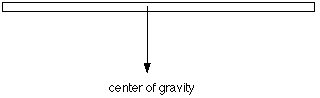A moment or a torque is caused by a force acting on a body some distance from the center of gravity. Its formula is:

torque = force x moment arm

where the force is always acting perpendicular to the moment arm. In the diagram below, item A is at a distance X from the fulcrum or pivot point, while item B is at distance Y from the fulcrum. If the system shown is balanced, then the product of A and X must equal the product of B and Y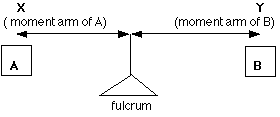The product of the weight of item A and its distance from the pivot point (X) produces a counterclockwise torque and the product of the weight of item B and its moment arm Y produces a clockwise torque. When the clockwise torques equal the counter clockwise torques, the airplane or object is balanced along that plane. in an airplane, any moment in front of the center of gravity is considered a negative value, having a negative moment arm, while and torque behind the center of gravity is considered to be a positive value with a positive moment arm.

Finding the balance point: Given the following setup below, where the length of the beam is 80 inches long but weightless. if we wish to find the balance point of this system, or where to locate the fulcrum, we first must set up an arbitrary zero reference point. This can be located anywhere on the line of the beam. For this example, we will choose the zero reference point to be at A.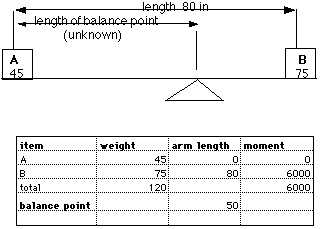By constructing a simple chart of weight, moment arms and moments as shown above, and placing the values in each space. Item A has a weight of 45 pounds. Since the zero reference point was selected at A, its arm length is 0. Zero times 45 is 0, so A's torque or moment is zero. B weighs 75 pounds and its arm length is 80 inches. Since 80 times 75 equals 6000 inches-pounds, B has a torque of 6000 inches-pounds. The total weight is 120 pounds and the total moment 6000 inches-pounds, the balance point is found by dividing the total moment by the total weight. This value is 50 inches located to the right of point A or since 80 - 50 = 30, it is also 30 inches to the left of B.

Checking these values, letting the moment arm of A be negative, then

A's torque = force x distance = 45 pounds x -50 inches = -2250 inches-pounds

B's torque = force x distance = 75 pounds x 30 inches = 2250 inches -pounds

Since the counterclockwise torque (-2250) and the clockwise torque (2250) sum to zero, the beam will balance at the point located 50 inches to the right of point A.

Problem:

1. Given the following figure below, where A has a weight of 168 pounds and B has a weight of 272 pounds, and the weightless beam is 120 inches long. Locate the center of gravity where the fulcrum should be placed.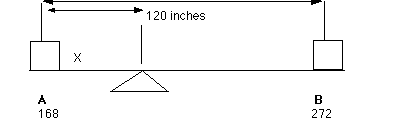2. Given the length of the weightless beam to be 120 inches, and the weight at A to be 600 pounds with the center of gravity to be located 4 feet to the right of A. Find the weight necessary to produce equilibrium at B.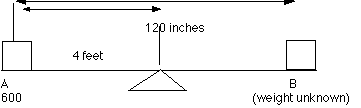Extension:
An aircraft that has additional equipment installed on the plane or replacements made by technicians needs to have the information about its center of gravity updated.

Table for question 1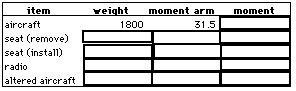1. An aircraft of known weight and center of gravity location is going through alterations. A 30-pound seat located at 72 inches with a 20-pound seat whose arm will be 73.5 inches. Also, a radio of 30-pounds is to be installed at 30 inches. Find the new center of gravity of the aircraft and complete the above table.

2. An aircraft with an empty weight of 1,500 pounds and a center of gravity (empty) of 28.4 inches was altered as follows:
1. Two seats weighing 12 pounds each, located at 65.5 were removed.

2. A structural modification increasing the weight by 28 pounds was made at 73 inches.

3. A seat and safety belt weighing 30 pounds were installed at 70.5.

4. radio equipment weighing 25 pounds was installed at 85 inches.

Find the following information: the new empty weight is____________,
and the new location of the CG is__________.+ Inspector General Hotline + Equal Employment Opportunity Data Posted Pursuant to the No Fear Act + Budgets, Strategic Plans and Accountability Reports + Freedom of Information Act + The President's Management Agenda + NASA Privacy Statement, Disclaimer, and Accessibility CertificationEditor: Tom Benson NASA Official: Tom Benson Last Updated: May 13 2021 + Contact Glenn